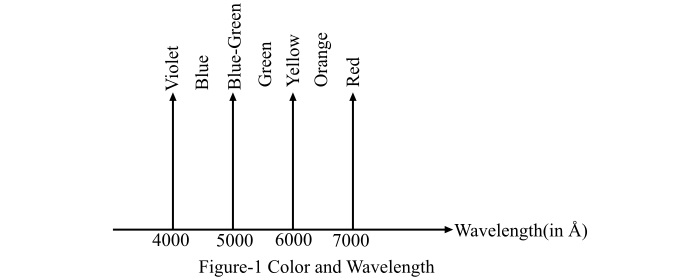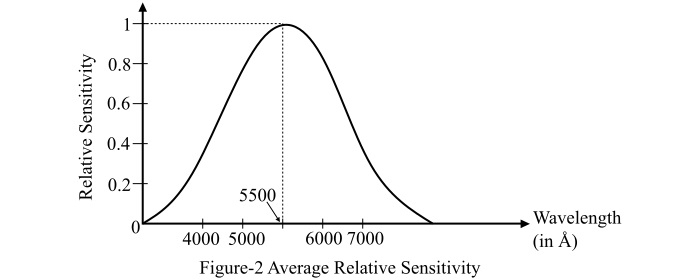# Common Terms Used in Lighting and Illumination

## What is Illumination?

The luminous flux received by the surface per unit area is known as illumination. Illumination is denoted by the letter ‘E’ and is measured in lux or lumen per square meter or meter candela or foot candela.

Mathematically, illumination is given by the formula,

$$\mathrm{\mathit{E}\:=\:\frac{\mathrm{Luminous\:Flux}}{\mathrm{Area}}}$$

Practically, the illumination deals with the selection, design, installation and maintenance of the artificial sources of light used in practice.

In this article, let's make sense of some of the common terms used in illumination.

## Color & Wavelength

The energy radiation of the heated body is of one color (or monochromatic), that means, the radiation of only one wavelength emits a specific color. The wavelength of visible light varies from 4000 Å to 7500 Å.. The wavelengths and the corresponding colors of the radiation are shown in Figure-1.## Relative Sensitivity

The reactive power of human eye to the light waves of different wavelengths varies from person to person and also varies with age. The human eye is most sensitive for wavelength of 5500 Å. Therefore, the relative sensitivity corresponding to 5500 Å is taken as unity. The average relative sensitivity is shown in Figure-2. The relative sensitivity at any particular wavelength is known as relative luminous factor, denoted by $\mathit{k_{\mathit{\lambda}}}$.## Light

The radiant energy from a hot body that produces the visual sensation to the human eye is known as light. Light is expressed in lumen-hours.

## Luminous Flux

The energy in the form of light waves radiated per second from a luminous body is termed as luminous flux. The luminous flux is denoted by the symbol ‘$\phi$’ and is measured in lumens.

## Plane-Angle

An angle subtended at a point in a plane by two converging lines is called a plane angle. It is denoted by symbol ‘$\theta$’ and is measured in degree or radians.Mathematically, the plane-angle is given by,

$$\mathrm{\mathrm{Plane\:angle} ,\theta \:=\:\frac{\mathrm{Arc\:length}}{\mathrm{Radius}}}$$

## Solid Angle

An angle subtended at a point in a space by an area, i.e., the angle enclosed in the volume formed by numerous lines lying on the surface and meeting at a point is called the solid angle. The solid angle is denoted by symbol $‘\omega’$ and is measured in steradian.Mathematically, it is given by,

$$\mathrm{\mathrm{Solid\:angle} ,\omega \:=\:\frac{\mathrm{Area}}{\mathrm{Radius^{\mathrm{2}}}} }$$

## Lumen

Lumen is the unit of luminous flux. Lumen is defined as the total luminous flux emitted by a source of one candle power for unit solid angle in all the directions.

Mathematically,

$$\mathrm{\mathrm{Lumen}\:=\:\mathrm{Candle\:power\:of\:source}\:\times \:\mathrm{solid\:angle}}$$

$$\mathrm{\Rightarrow \mathrm{Lumen}\:=\:\mathit{CP}\:\times \omega }$$

## Candle Power

The total luminous flux lines emitted by a source per unit solid angle is known as candle power of that source. It is measured in lumen per steradian.

Mathematically,

$$\mathrm{\mathit{CP}\:=\:\frac{\mathrm{Lumen}}{\omega }}$$

## Lux or Meter Candle

It is the unit of illumination. The illumination of the inside of a sphere of radius 1 m and a source of 1 candle power is fitted at the center of the sphere is known as lux or meter candle.

## Foot Candle

Foot candle is also the unit of illumination. It is defined as the illumination of the inside of a sphere of radius 1 foot and a source of 1 CP is fitted at the center of the sphere.